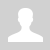Python. Lambda function. map(), filter() functions. (en)

11.3.2.1 write code in a programming language using lambda functions
11.3.2.2 determine the result of a lambda function

# Python. Lambda function. map(), filter() functions.

Lambda function

Often, we want to use a very simple function as an argument for higher-order functions. Moreover, such a function is often needed in the program only in one place, so it does not even need to have a name.

The Lambda operator can be used to create anonymous functions. They do not need to be isolated as a separate function and can be used as an argument to another function.

Unnamed (anonymous) functions can be created with a statement:

lambda <arguments>: <expression>

For example, the next function

```def mult(x, y):     return x * y```

can be written as:

`mult = lambda x, y: x * y `

Example

```mult = lambda x, y: x * y print(mult(3, 5)) # => 15 print(mult(5, mult(2, 3))) # => 30```

map() function

The map() function returns a map object (which is an iterator) of the results after applying the given function to each element of the given iteration (list, tuple, etc.)

The map function can be written using the following statement:

map(<function>, <iterable object>)

Examples

Task. The map function can be used when entering two numbers on the same line. Each string that we get in the list with the help will be converted to an integer.

We can use the next code:

```lst = input().split()  n1 = int(lst) n2 = int(lst)```

Or change for the next code using the map function:

`n1, n2 = map(int, input().split()) # if we input line 5 and 7, then n1 = 5, n2 = 7`

If we want to increase each number from the range from 5 to 10 by 2, then we use the map function and pack the resulting values into a list.

```increased_numbers = list(map(lambda x: x + 2, range(5, 11))) print(increased_numbers) # [7, 8, 9, 10, 11, 12]```

filter() function

Filter() is a built-in Python function. You can apply a filter function to an iterable, such as a list or dictionary, and create a new iterator. This new iterator can filter out specific elements very efficiently based on the condition you provide.

The filter function can be written using the following statement:

filter(<function>, <iterable object>)

Examples

Task. Print all integers from 10 to 50 that are divisible by 10.

```round_numbers = list(filter(lambda x: x % 10 == 0, range(10, 51)) print(round_numbers) # [10, 20, 30, 40, 50]```

Task. Using the filter function, remove from the list only those words whose length is greater than five

```words = ["computer", "desk", "keyboard", "pencil", "mouse"] print(list(filter(lambda word: len(word)>5, words))) # ["computer", "keyboard", "pencil"]```

Task. Print the squares of all integers between 0 and 20 that are divisible by 3.

```squares_numbers = list(map(lambda x: x ** 2, filter(lambda x: x % 3 == 0, range(21)))) # filter determines numbers that divide by 3  print(squares_numbers) # output [0, 9, 36, 81, 144, 225, 324]```

Questions:

1. Which function has no name? Explain its structure.
2. Why do we use the lambda function instead of the regular function?

Exercises:

Ex. 1 Lambda functions